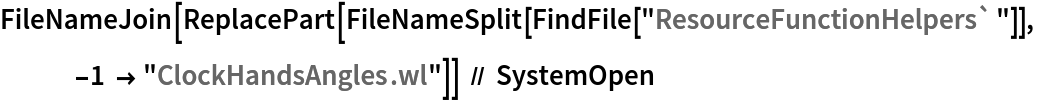Function Repository Resource:

# ClockHandsAngles

Compute the angle between the hands of a clock

Contributed by: Wolfram|Alpha Math Team
 ClockHandAngles[timeSpec] returns an association of information for the angle between the hands of a clock at the given time. ClockHandAngle[timeSpec,unit] returns the clock information in the angular units specified by units.

## Details and Options

The input units can be "Degrees" or "Radians".
The association returned has the following keys:
 "HourMinuteAngle" angle between hour and minute hands of a clock "HourSecondAngle" angle between hour and second hands of a clock "MinuteSecondAngle" angle between minute and second hands of a clock "ClockImage" displays clock set to the current time
The input timeSpec can be a DateObject, TimeObject or any input supported by TimeObject.

## Examples

### Basic Examples (2)

Compute the angle between clock hands at 2:20:

 In:=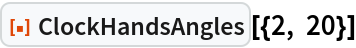Out=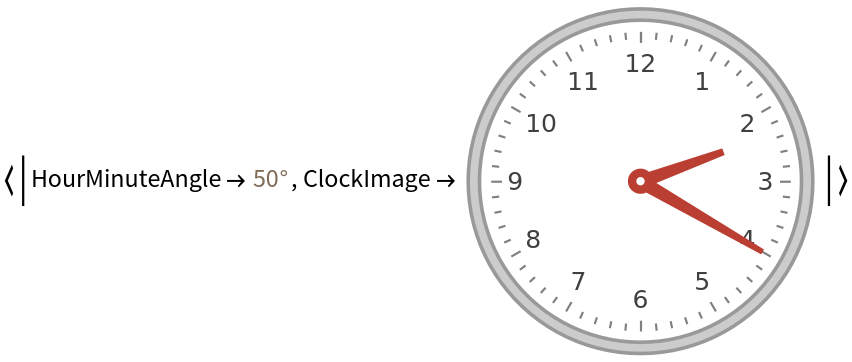Return only the angular Quantity:

 In:=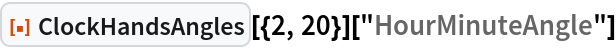Out=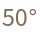Compute the angles at time 6:20:20:

 In:=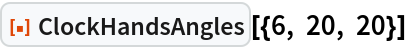Out=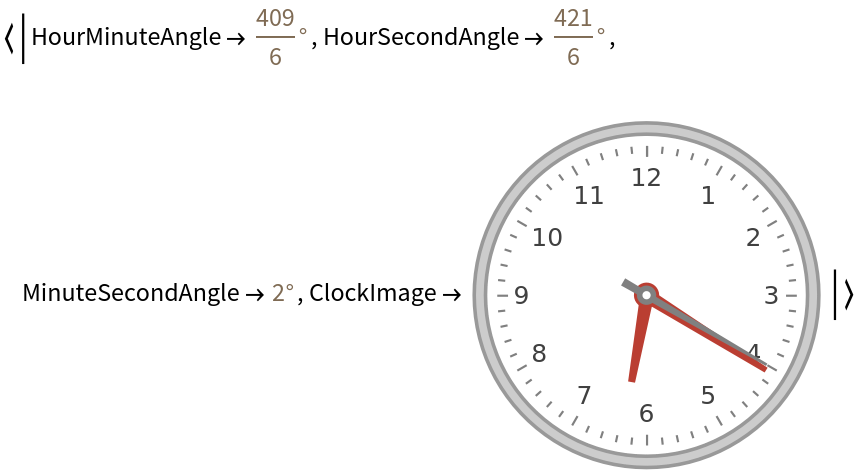### Scope (2)

Compute the angles of the clock hands at the current time:

 In:=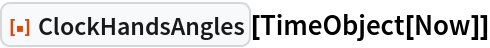Out=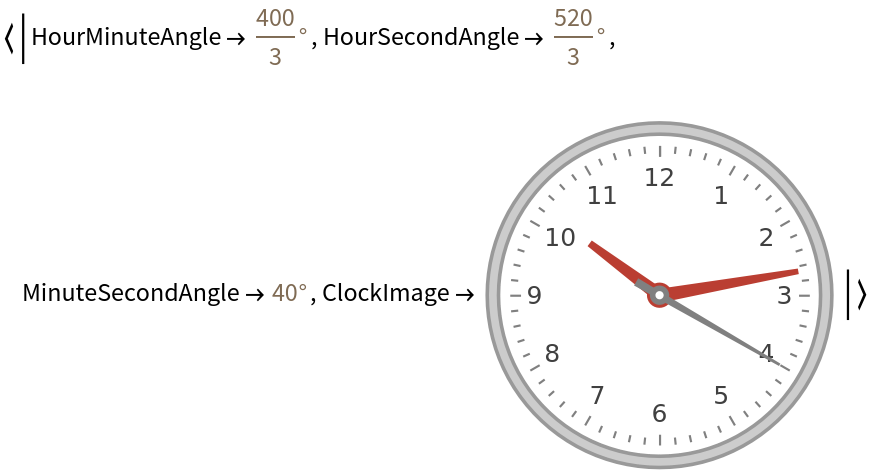Compute the angle between clock hands in radians:

 In:=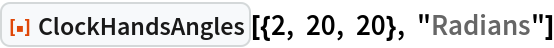Out=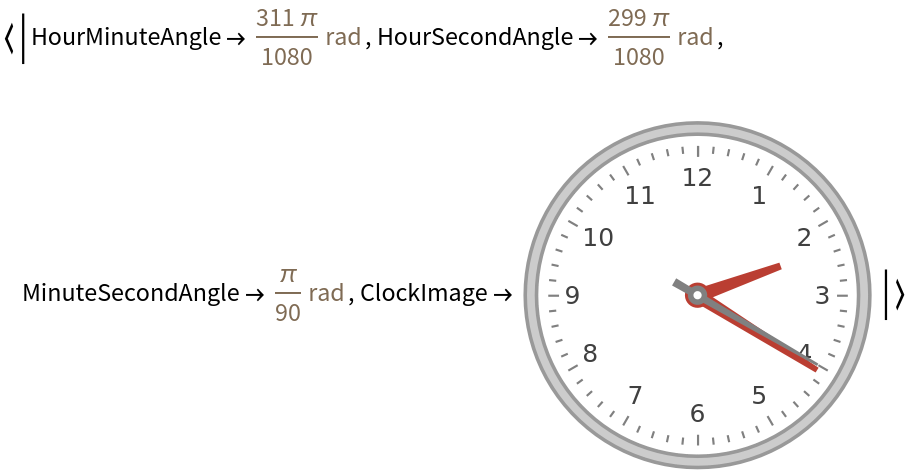## Publisher

Wolfram|Alpha Math Team

## Version History

• 2.0.0 – 23 March 2023
• 1.0.0 – 18 September 2020

## Author Notes

To view the full source code for ClockHandsAngles, run the following code:

 In:=